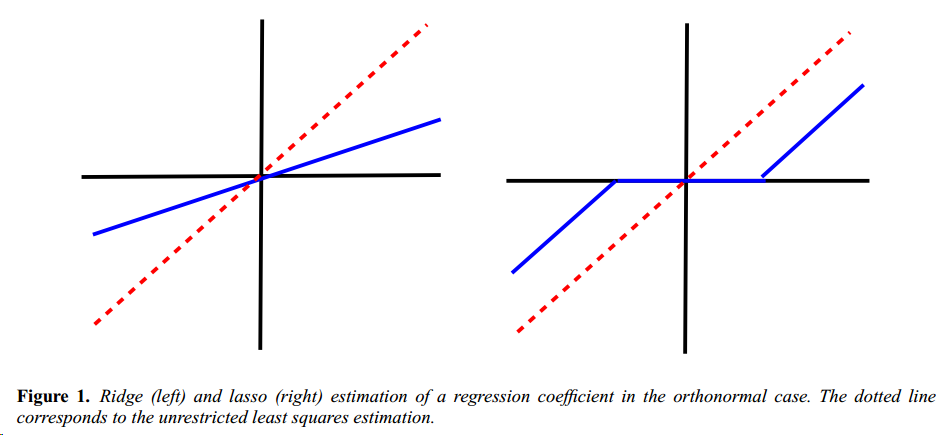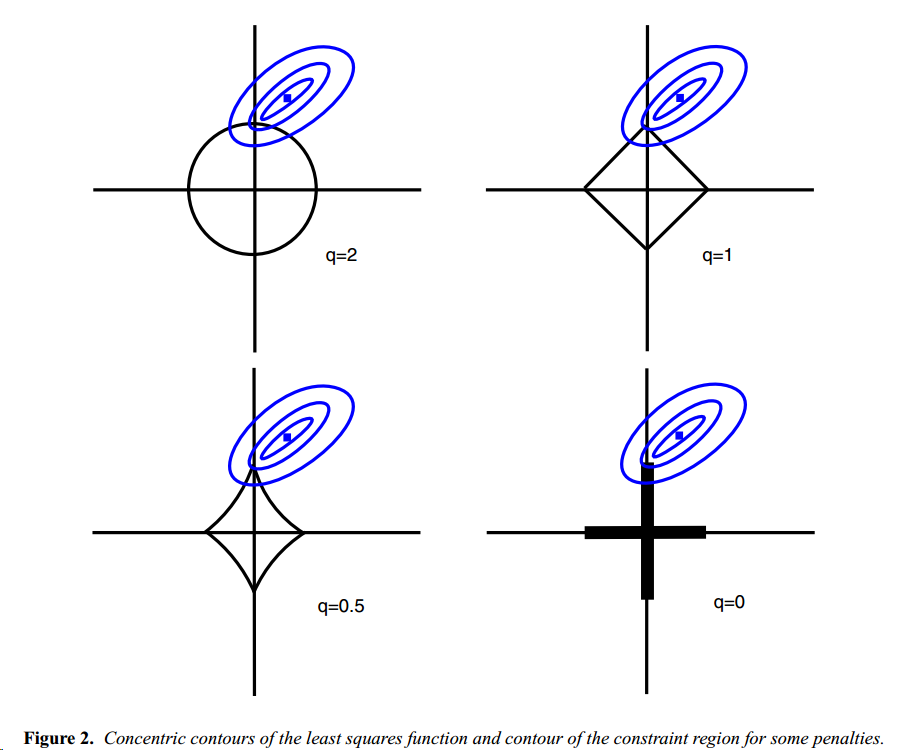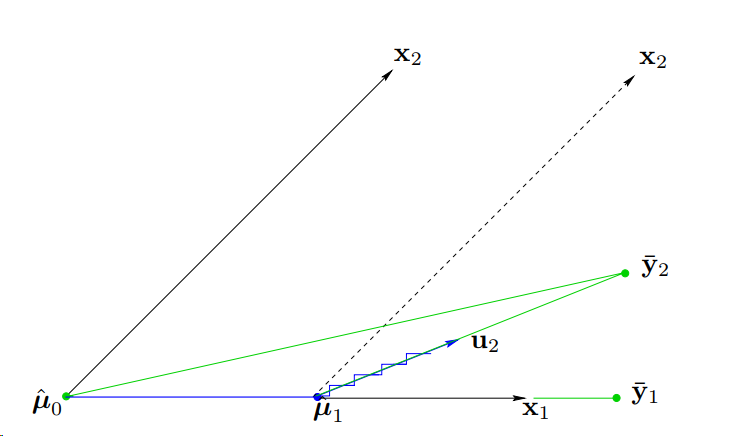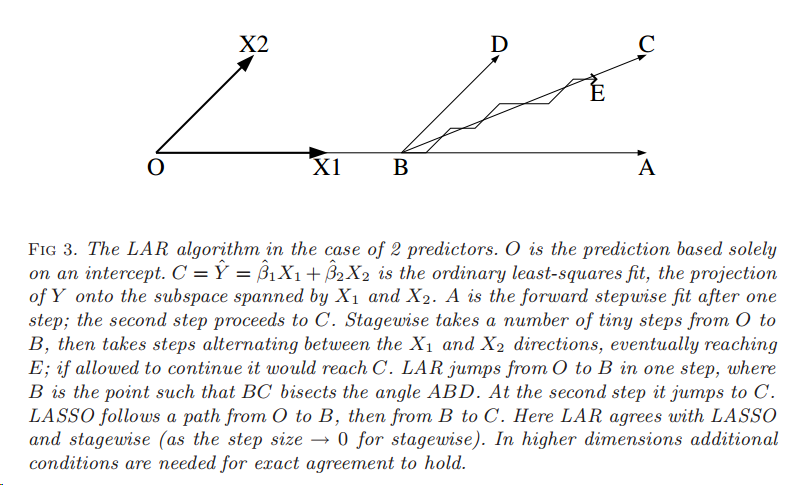# 闲话Variable Selection和Lasso

## Lasso

$y=X\beta+\epsilon$

\begin{aligned} \hat{\beta}=\underset{\beta}{\arg\min}\|y-X\beta\|^2_2 &&\text{s.t. }\|\beta\|_1\leq s \end{aligned}

$\hat{\beta}=\underset{\beta}{\arg\min}\|y-X\beta\|^2_2+\lambda\|\beta\|_1$

$\hat{\beta_j}=\text{sign}(\hat{\beta_j^{\text{ls}}})(\hat{\beta_j^{\text{ls}}}- \lambda)_+,j\in{1,\cdots,p}$

$\hat{\beta_j}=\hat{\beta_j^{\text{ls}}}/(1+\lambda),j\in{1,\cdots,p}$1

$\text{AIC}=\log\|\hat{y}-y\|^2_2+2\text{df}$

\begin{aligned} \text{AIC}=2k-2\ln(\hat{L})\\ \text{BIC}=\ln(n)k-2\ln(\hat{L}) \end{aligned}2

## lasso和robust regression

lasso某种程度上也是一种鲁棒性回归，

$\hat{\beta}=\underset{\beta}{\arg\min}\{\max_{z\in\theta}\|y-(X+Z)\beta\|_2\}$

## LAR34

Stepwise的方式是O->A->C，Stagewise的方式是O->B->E->C，注意其中O->B和B->E都是多步尝试后到达，LAR则是O->B->C，都是选择很合适的位置，其中BC平分角DBA，因为LAR选择时会在BA，BD同样相关的情况（LAR选择策略，一个step前进至两个变量和预测值具有相同相关性的位置）。以上都是在比较理想的情况，大部分高维的情况下都需要满足一定条件才会收敛。

LAR有几个比较remarkable的性质：

• 速度快，当$p < n$时，需要$O(p^3+np^2)$次最小二乘拟合的计算
• 可以用于拟合Lasso和stagewise模型
• 可以使用简单的统计标准来选择步数，如$C_p$

$C_p=(1/\hat{sigma}^2)\sum^n_{i=1}(y_i-\hat{y_i})^2-n+2k$

## Pathwise coordinate descent for lasso

[3, 4]中提到利用坐标下降的方式求解Lasso，求解lasso的原问题等价于求解

$\min_\beta\frac{1}{2}(\beta-\hat{\beta})^2+\gamma|\beta|$

$\frac{df}{d\beta}=\beta-\hat{\beta}+\gamma=0$

\begin{aligned} \hat{\beta}^{\text{lasso}}(\gamma)&=S(\hat{\beta},\gamma)=sign(\hat{\beta})(|\hat{\beta}|-\gamma)_+ \\ &=\begin{cases} \hat{\beta}-\gamma,&&\hat{\beta}>0, \gamma<|\hat{\beta}|\\ \hat{\beta}+\gamma,&&\hat{\beta}<0, \gamma<|\hat{\beta}|\\ 0,&&\gamma>|\hat{\beta}|\\ \end{cases} \end{aligned}

$f(\tilde{\beta})=\frac{1}{2}\sum^n_{i=1}(y_i-\sum_{k\neq j}x_{ik}\tilde{\beta}_k-x_ij\beta_j)^2+\gamma\sum{k\neq j}|\tilde{\beta}_j|+gamma|\beta_j|$

$\tilde{\gamma}\leftarrow S(\sum^n_{i=1}x_{ij}(y_i-\tilde{y}_i^{(j)}),\gamma)$

## Lasso的贝叶斯解释

$\pi(\beta|\sigma^2)=\prod_{j=1}^{p}\frac{\lambda}{2\sigma}\exp\frac{-\lambda|\beta_j|}{\sigma}$

## Lasso改进

 提出一种adaptive lasso，给每个变量不同的重要性

$\hat{\beta}=\underset{\beta}{\arg\min}\|y-X\beta\|^2_2+\lambda\sum_{j=1}^{p}w_j|\beta_j|$

$N\geq p$时，权重计算为

$w_j=\frac{1}{|\hat{\beta}_j^{\text{ls}}|^\gamma},\gamma\geq 0$

$N < p$时，权重通过计算最小二乘估计时的最小正则项得到。中表明adaptive lasso在变量选择方面，比其他lasso模型更优。

里面提出elastic net，同时结合了L1和L2正则项

$\hat{\beta}=\underset{\beta}{\arg\min}\|y-X\beta\|^2_2+\lambda(\alpha\|\beta\|_1+(1-\alpha)\|\beta\|_2^2)$

## Reference

 Tibshirani, R. (1996). Regression Shrinkage and Selection via the Lasso. J. Roy. Stat. Soc. Ser. B, 58(1), 267–288.

 Efron, B., Johnstone, I., Hastie, T. & Tibshirani, R. (2004). Least Angle Regression. Ann. Statist., 32(2), 407–499

 Friedman, Jerome, et al. “Pathwise coordinate optimization.” The Annals of Applied Statistics 1.2 (2007): 302-332.

 Wu, Tong Tong, and Kenneth Lange. “Coordinate descent algorithms for lasso penalized regression.” The Annals of Applied Statistics 2.1 (2008): 224-244.

 Meinshausen, N. (2007). Lasso with Relaxation. Comput. Stat. Data Anal., 52(1), 374–393.

 Radchenko, P. & James, G. M. (2008). Variable Inclusion and Shrinkage Algorithms. J. Amer. Statist. Assoc., 103(483), 1304–1315.

 Zou, H. (2006). The Adaptive Lasso and Its Oracle Properties. J. Amer. Statist. Assoc., 101(12), 1418–1429.

 Zou, H. & Hastie, T. (2005). Regularization and Variable Selection via the Elastic Net. J. Roy. Stat. Soc. Ser. B, 67(2), 301–320

 Bach, F. R. (2008a). Bolasso: Model Consistent Lasso Estimation Through the Bootstrap. 15th International Conference on Machine Learning; 33–40.

 Chatterjee, A. & Lahiri, S. N. (2011). Bootstrapping Lasso Estimators. J. Amer. Statist. Assoc., 106(494), 608–625.

 Vidaurre, Diego, Concha Bielza, and Pedro Larrañaga. “A survey of L1 regression.” International Statistical Review 81.3 (2013): 361-387.

 Tibshirani, Robert. “Regression shrinkage and selection via the lasso: a retrospective.” Journal of the Royal Statistical Society: Series B (Statistical Methodology) 73.3 (2011): 273-282.

 Hesterberg, Tim, et al. “Least angle and ℓ1 penalized regression: A review.” Statistics Surveys 2 (2008): 61-93.

 Fan, Jianqing, and Runze Li. “Variable selection via nonconcave penalized likelihood and its oracle properties.” Journal of the American statistical Association 96.456 (2001): 1348-1360.

 Candes, Emmanuel, and Terence Tao. “The Dantzig selector: Statistical estimation when p is much larger than n.” The Annals of Statistics 35.6 (2007): 2313-2351.